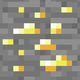# Unresolved Detail In Plotted Equations

Sometimes the calculator detects that an equation is too complicated to plot perfectly in a reasonable amount of time. When this happens, the equation is plotted at lower resolution.

281 out of 311 found this helpful
Have more questions? Submit a request

•D35M05BR34K4G3MA5T3R

That's Desmos Art ;)

•Tanksear Industries
•Tanksear Industries

\sin\left(\frac{x}{y}\right)\le\tan\left(\sqrt{\frac{y}{x}}-\frac{\log\left(\frac{x}{y}\right)}{\sqrt{x^y}}+y^{\frac{x}{x^{\frac{y}{x^y}}}}\right)

Try zooming in on some parts of this one.

•Lking22

\frac{\cos\left(\frac{x}{y}\right)}{\tan\left(\frac{x}{y}\right)}!<\left(x\cdot y\right)

•Lking22

\frac{\left(\frac{\frac{\sin\left(x\right)^{-1}}{\tan\left(y\right)^{-2}}}{\sin\left(x\right)}\right)}{\sin\left(x\tan\left(y\right)\right)}>\frac{\left(\frac{\left(\frac{\tan\left(xy\right)^{-3}}{\sin\left(y\right)^{-2}}\right)\sin\left(yx\right)}{\frac{\cos\left(x\right)^{-1}}{\cos\left(y\right)}}\right)}{\tan\left(xy\right)!}

•Tanksear Industries
•Tanksear Industries

\sin\left(x^{y!}\right)\le\sqrt{\tan\left(xyx\right)}

•Lking22

\frac{\sin\left(x\right)}{\cos\left(y\right)}\ge\log\left(\frac{x}{y}\right)^{\frac{x}{y}}\sin\left(y\right)!

•Lking22

\sin\left(x\right)\sec\left(xy\right)^{\frac{x}{y}}\le\log\left(y\right)^{\frac{y}{x}}\cos\left(xy\right)

•26ej0225

Talk about taking it to a whole new level.

(x^2-y)!<=2

•12044289

https://www.desmos.com/calculator/n0d3p6gczv

Zoom in a few times to get that nice fine detail

Then wait for it to load

•Tanksear Industries

https://www.desmos.com/calculator/xm0nyvw0oc

I went back to my roots. Specifically the \operatorname{mod}\left(\operatorname{mean}\left(\sin x,\cos x,\sqrt{\sqrt{x-y}}\right),\operatorname{median}\left(\sin x,\cos x,\sqrt{\sqrt{x-y}}\right)\right) of x and the \sqrt[\operatorname{mod}\left(\sqrt{\sqrt{x-\operatorname{mod}\left(\sqrt{x},yx^x\right)}},\sqrt{x}-\operatorname{mean}\left(\operatorname{median}\left(y,xy,\sqrt{\sqrt{yx}}\right),\sqrt{\sqrt{x-yx}},y^x,x-y^x,\frac{xx}{yx^{yx}},x\right)\right)] root of xy.

•Bennettemickley

y=x^y

•Tanksear Industries

\frac{y}{\sin y}+y\le\frac{x}{\sin x}-xy

Modern Art

•Sun Shengkun

You can try y=\csc\left(\cot\ \left(x\frac{f\left(x\right)}{\frac{xy\left(\cot\ x\right)}{y}}\right)\right) it look like sound waves

•Sun Shengkun

this one takes it to a whole new level

\cot\left(\frac{xy}{\frac{\csc\left(xy\left(\cot x\right)\right)}{\cot\ \frac{xyxy}{12}}}\right)+\sec\left(\frac{xy}{\frac{\cot\left(xy\right)}{x}}\right)=y

•Sun Shengkun
•Sun Shengkun
•Tanksear Industries
•Tanksear Industries
•Tanksear Industries
•Thecubicalguy1

Found a new one: x^{\log y}=y^{\log x}

•Evin Liang

(d/dx)x!!=tan(y)^2

•Tanksear Industries
•Tanksear Industries

\sin\left(x\right)^y\le\sin\left(x\right)^x

Explore this one when x>0 and y>0 to find some weird stuff.

•Hey bud,

The reason it isn't working because with quadratic functions you can't have two "=" sings.

If I'm wrong please let me know!

•For some reason, this works???

y=\left(\cos\left(x\right)+\cos\left(y\sin\left(\frac{\pi}{5}\right)+x\cos\left(\frac{\pi}{5}\right)\right)+\cos\left(y\sin\left(\frac{2\pi}{5}\right)+x\cos\left(\frac{2\pi}{5}\right)\right)+\cos\left(y\sin\left(\frac{3\pi}{5}\right)+x\cos\left(\frac{3\pi}{5}\right)\right)+\cos\left(y\sin\left(\frac{4\pi}{5}\right)+x\cos\left(\frac{4\pi}{5}\right)\right)\right)

•Evin Liang
•23ccamarena

\tan\left(\frac{x}{yx}\right)^{y^2}=\left(\frac{\sqrt{\cos\left(\frac{x}{y}\right)}}{y^x}\cos\left(\frac{xy}{y^x}\right)\right)^{y^{x\cdot y}}

•